Share

# Find the Particular Solution of the Differential Equation (X - Y) Dy/Dx = (X + 2y) Given that Y = 0 When X = 1. - CBSE (Science) Class 12 - Mathematics

ConceptMethods of Solving First Order, First Degree Differential Equations Homogeneous Differential Equations

#### Question

Find the particular solution of the differential equation (x - y) dy/dx = (x + 2y) given that y = 0 when x = 1.

#### Solution 1

dy/dx = (x + 2y)/(x - y)

Putting y = Vx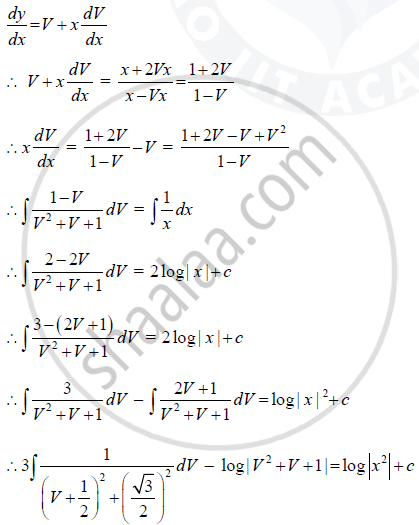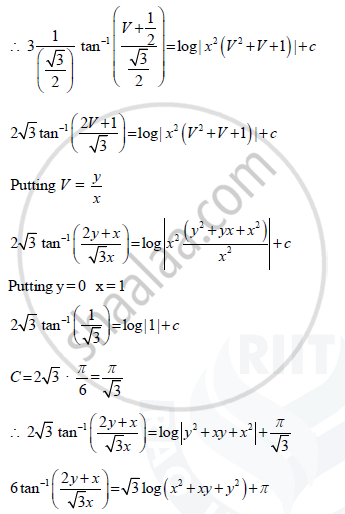#### Solution 2

The given differential equation is

(x - y) dy/dx = x + 2y

=> dy/dx = (x + 2y)/(x - y)

This is a homogeneous differential equation.

Putting y=vx and dy/dx = v + x dy/dx, we get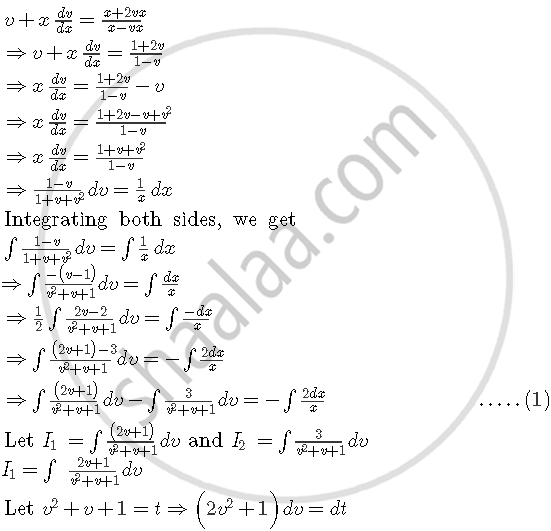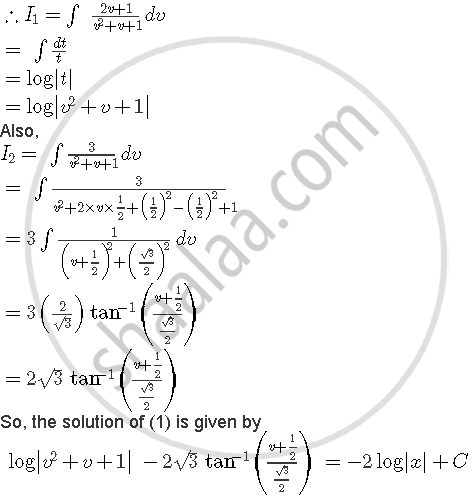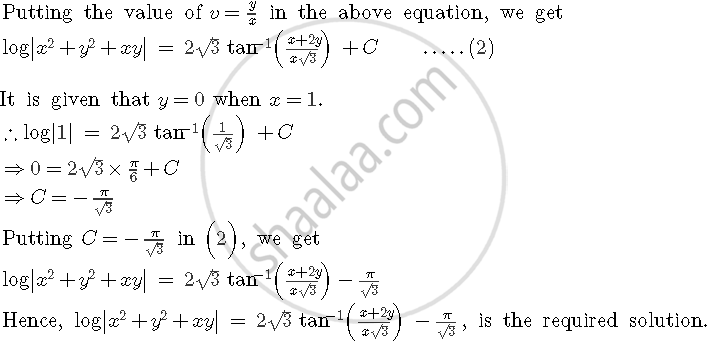Is there an error in this question or solution?

#### APPEARS IN

Solution Find the Particular Solution of the Differential Equation (X - Y) Dy/Dx = (X + 2y) Given that Y = 0 When X = 1. Concept: Methods of Solving First Order, First Degree Differential Equations - Homogeneous Differential Equations.
S